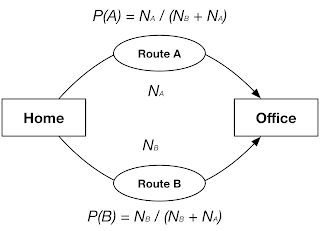## Monday, 11 January 2016

### The effect of repetition in learning

Our habits are strange, the more you do them the more it's difficult for you to get out of them. In a sense, it seems like some simple probability rules are controlling our habits, as you use them more the chance of doing them again gets increased and in order to get rid of them you have to either not repeat them or you have to do something else more.

Let's get back to our previous example, "route to work".  Look at the picture our subject usually uses route A or B to get to the office, every time he takes one of these routes we increase a counter like NA or NB which at any time shows the number of the times the subject has taken the route.Learning model when you have 2 options to choose from

So the probability of taking any of these routes at any time will be like the followings:

P(A) = NA / (NA + NB)
P(B) = NB / (N+ NB)

Now if the subject takes for example Route A more than B, the P(A) gets higher and higher, in this simple model if for 500 of samples the subject takes Route A, 425 times and Route B 75 times, the next time he would choose Rout A with probability of 425/500 or 0.85 and Route B with probability of 0.15.

For this subject using Rout A will be the most probable choice until the day the probability of choosing any of them equals to 0.5, in our example he/she must continue using Route B then in next n times of taking route B the probability will be 0.5, to find n we have to solve this simple equation:

(n + 75) / (500 + n) = 0.5   =>   n = 350

So after 350 times of continuously taking Route B, the probabilities will be:

P(A) = (425) / (500+350) = 0.5
P(B) = (75+350) / (500+350) = 0.5

From this point if he/she continues using Route B, gradually the chance of taking Route B gets bigger than Route A and we have to expect to see the subject mostly in Route B.

This simple example exactly shows how a simple habit or pattern can get learned or forgot, you surely experienced it in your life too when you want to not forget to brush your teeth after lunch, you have to do it more and more.

It is also the same idea but a bit complex in our catching the frisbee or ball by a dog or goalkeeper, the more they practice the easier for them to catch it. Although you have to consider that training a biological machine like human or an animal requires a more complicated model than what we discussed but in a nutshell, the idea is the same.